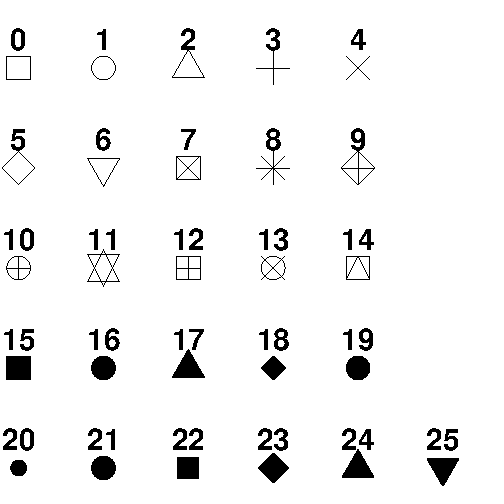# R 语言 – 绘图

## 绘制点

plot() 函数用于在图表中绘制点（标记）。

• 参数 1 指定 x 轴 上的点。
• 参数 2 指定 y 轴 上的点。

plot(1, 3)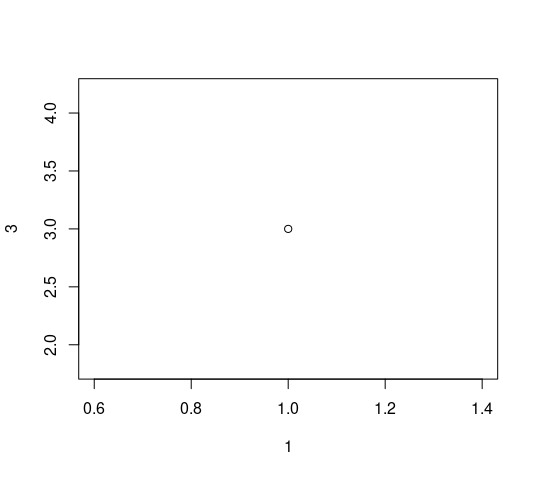## 绘制多点

plot(c(1, 8), c(3, 10))


plot(c(1, 2, 3, 4, 5), c(3, 7, 8, 9, 12))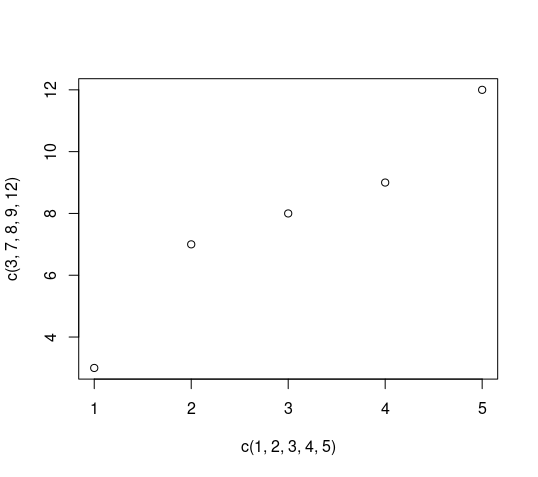x <- c(1, 2, 3, 4, 5)
y <- c(3, 7, 8, 9, 12)

plot(x, y)


## 点序列

plot(1:10)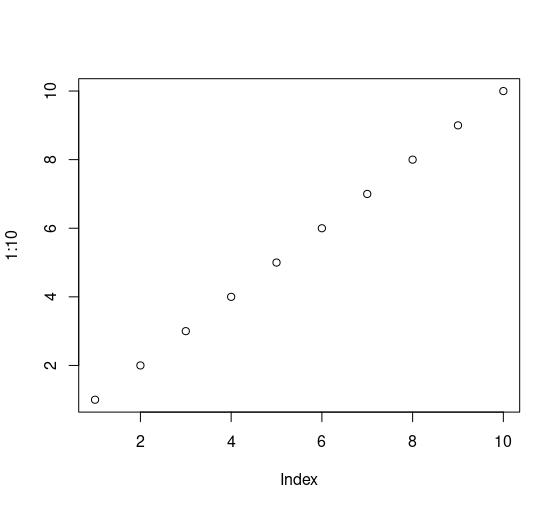## 绘制线条

plot() 函数还可以采用值为 ltype 参数来绘制一条线来连接图中的所有点。

plot(1:10, type="l")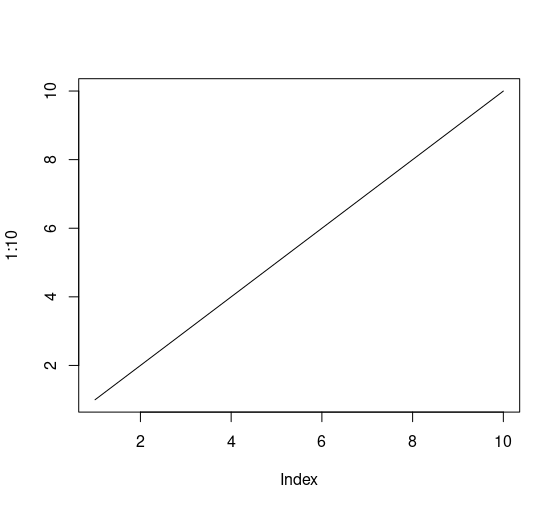## 绘图标签

plot() 函数还接受其他参数，例如 mainxlabylab，如果你想使用主标题和 x 轴和 y 轴的不同标签自定义图形。

plot(1:10, main="My Graph", xlab="The x-axis", ylab="The y axis")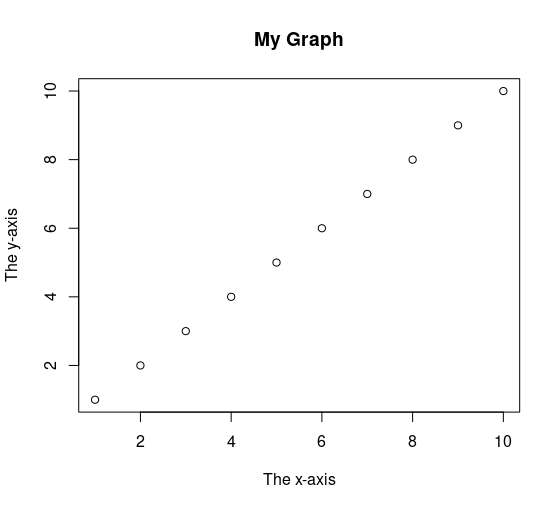## 图形外观

### 颜色

plot(1:10, col="red")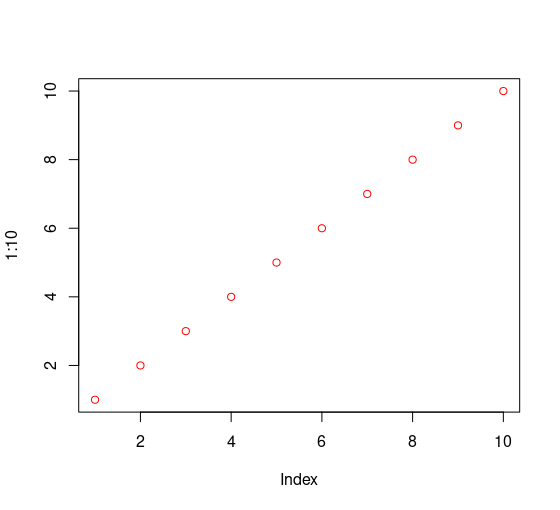### 大小

plot(1:10, cex=2)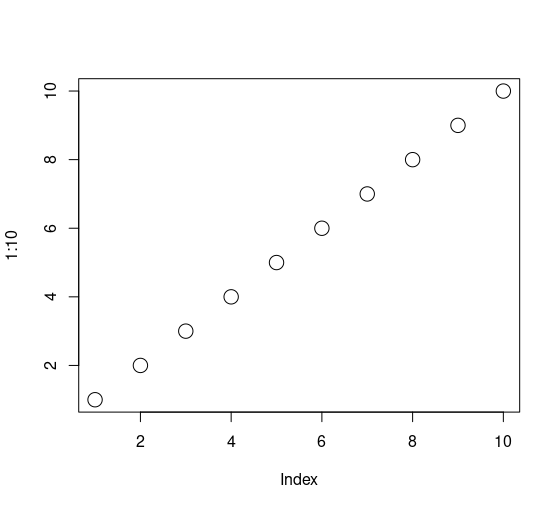### 形状

plot(1:10, pch=25, cex=2)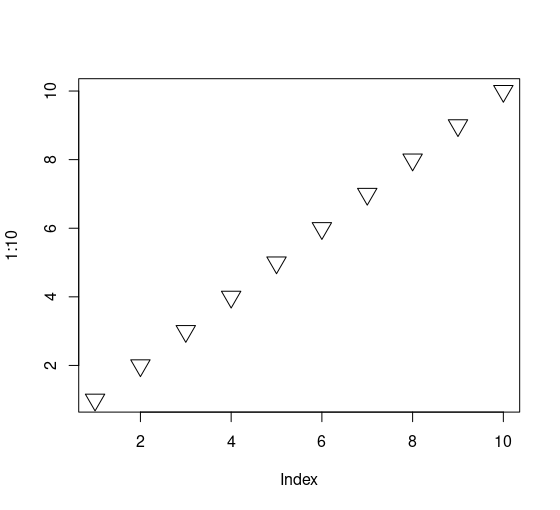pch 参数的取值范围为 0 到 25，这意味着我们最多可以选择 26 种不同类型的点形状，下图列出了所有形状。